# Linux下gcc编译器的使用

2020-02-29   374 次阅读

``````#include <stdio.h>
int main(){
printf("Hello world!");
return 0;
}
``````

### 第一种编译方式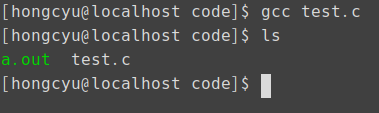a.out就是刚刚生成的可执行文件，现在我们要执行它。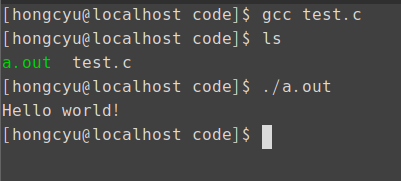gcc <filename.c>

### 第二种编译方式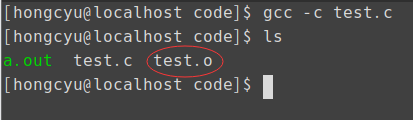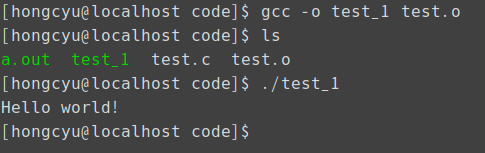1. gcc -c <filename.c>
2. gcc -o <filename_exe> <filename.o>

``````#include <stdio.h>
int main(){
int ret = 0;
int data = 100;
printf("Hello world!\n");

ret = sqrt(data);
printf("the sqrt = %d\n",ret);

return 0;
}
``````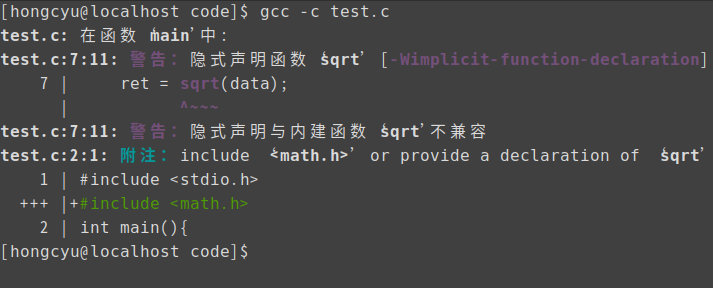``````#include <stdio.h>
#include <math.h>
int main(){
int ret = 0;
int data = 100;
printf("Hello world!\n");

ret = sqrt(data);
printf("the sqrt = %d\n",ret);

return 0;
}
``````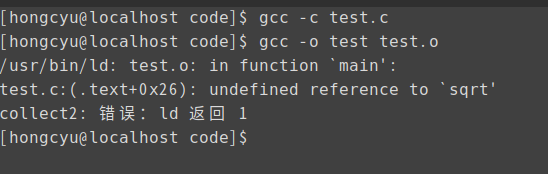`gcc -o test test.o -lm`(链接math.h不是-lmath而是-lm)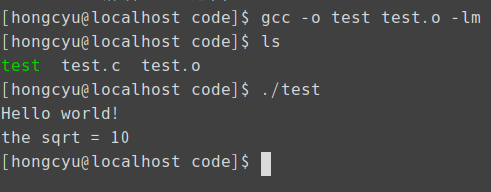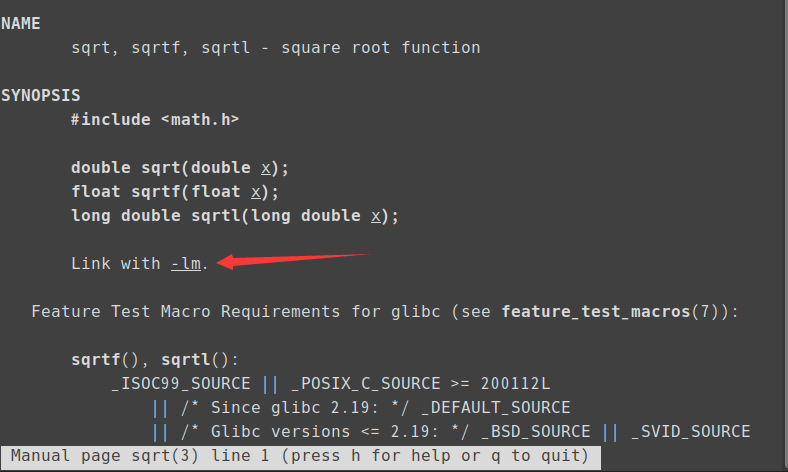### 第三种编译方式

`test.c`:

``````#include <stdio.h>
#include <math.h>
#include "myfunc.h"
int main(){
int ret = 0;
int data = 100;
int a = 0;
int b = 0;
printf("Hello world!\n");

ret = sqrt(data);
printf("the sqrt = %d\n",ret);

b = sub(1000,200);
printf("a = %d , b = %d \n",a,b);

return 0;
}
``````

`myfunc.c`:

``````int add(int x, int  y)
{
return (x+y);
}

int sub (int x, int y)
{
return (x-y);
}
``````

`myfunc.h`:

``````int add(int x,int y);
int sub(int x, int y);
``````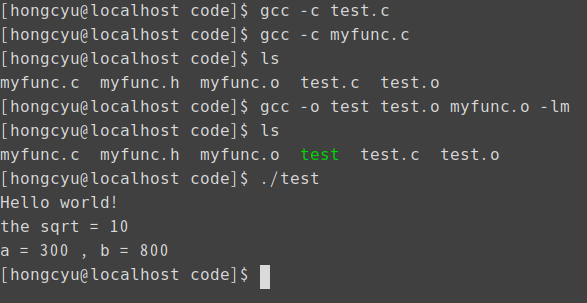tips：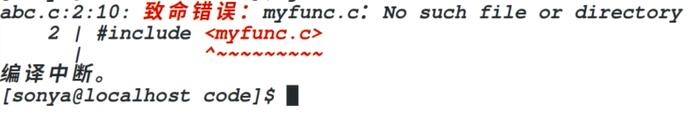1. gcc -c <filename1.c>
2. gcc -c <filename2.c> ...
3. gcc -0 <filename_exe> <filename.o> <filename2.o> ...... -l .....

### 第四种编译方式（makefile）

``````test:test.o myfunc.o
gcc -o test test.o myfunc.o -lm
test.o:test.c
gcc -c test.c
myfunc.o:myfunc.c
gcc -c myfunc.c
clean:
rm *.o
``````

tips:

`gcc,rm`等命令前加的是TAB （注意不要用空格代替制表符！）

makefile文件格式总结：

<模块名>:<生成所需资源文件>

<生成方法>

### gdb调试方法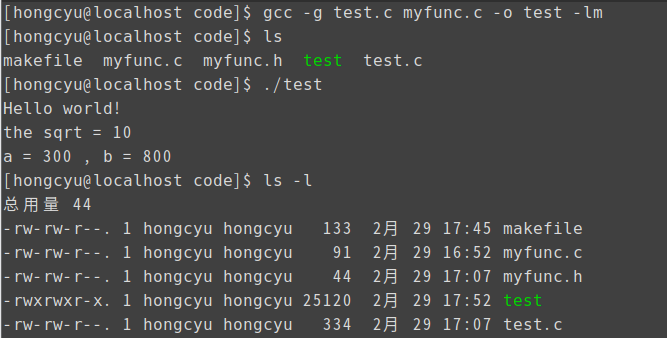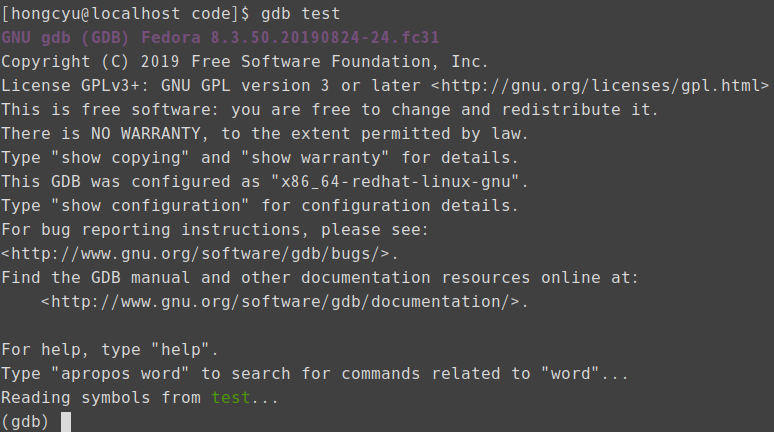1. list （查看10行的内容）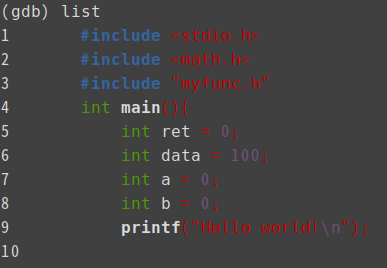2. list 10 (以第10行为中心上下共10行的内容)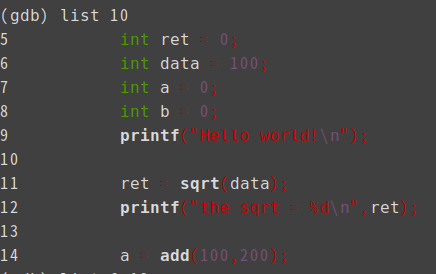3. list 6,12 (列出6到12行的内容)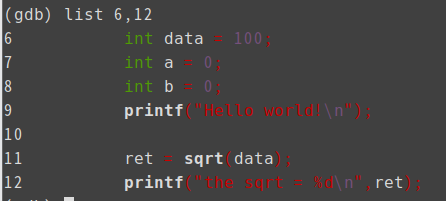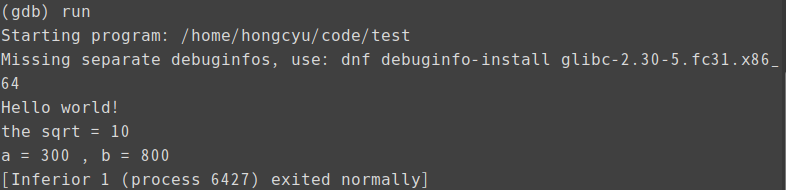1. 设置断点 break 行数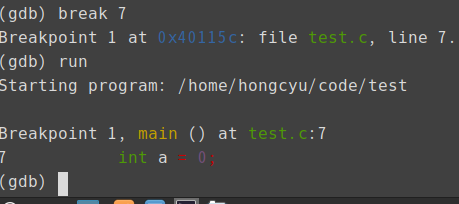2. 清除断点

采用`info break`查看断点信息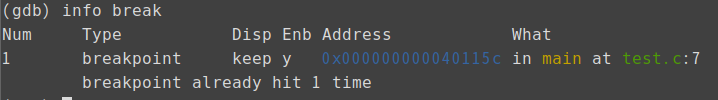输入 `delete breakpoint <Num>`即可删除对应编号的断点。

3. `print data` 即可查看当前赋的值

4. 单步运行

① step进入函数体的单步执行

② next 不进入函数体的单步执行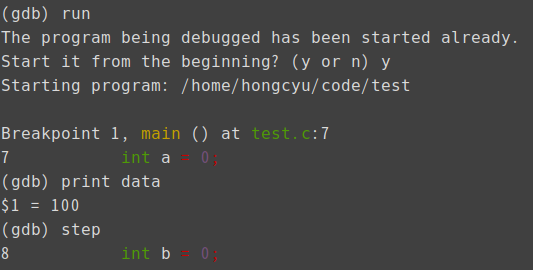5. 多步执行

`step <步数>`

`next <步数>`

gdb总结：

1. 生成： gcc -g <源文件> -o <执行文件> -l<库>

2. 进入调试 gdb <filename_exe>

3. list 显示十条语句

list n 以n为中心，显示十条语句

list m,n 显示从m到n的语句

4. break <行号> 设断点

5. info break 显示断点信息

6. delete breakpoint <编号>

7. enable/disable breakpoint <编号> 启用/禁用 断点

8. run 执行到断点

9. step进入函数体的单步执行

next 不进入函数体的单步执行

10. print <变量> 查看变量信息Coffee
••